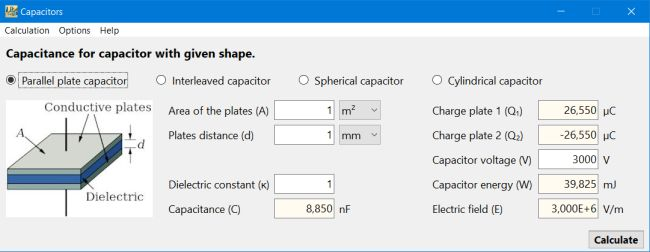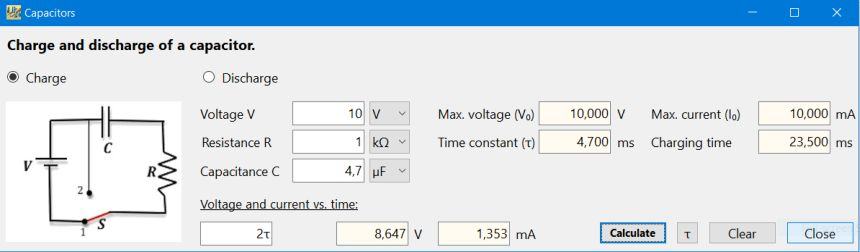# Computing: Free Pascal Programming

## Some common capacitor calculations.

Description: Simple electronics application, that may be used to do the following calculations:

• Calculation of the capacitance for a given capacitor shape (parallel plates, interleaved, spherical, cylindrical).
• Calculation of the capacitance for a given dielectric material (selectable from common material lists).
• Calculation of the total capacitance of a parallel or series circuit (2 to 4 capacitors).
• Calculation of all relevant values of a capacitor charge/discharge DC circuit (including, V and I calculation for a given time).
Application help as well as all formulas used by the application, included.

Note: If you need detailed calculations concerning the charge/discharge of a capacitor in a DC circuit, have a look at my Capacitor charge application, that draws graphs showing the voltage and the current as a function of time (application in French).

Free Pascal features: Showing/hiding form controls depending on situation. Re-arranging form controls during runtime. Loading images from file. Filling memos during runtime.

Screenshots: